American Journal of Theoretical and Applied Statistics
Volume 5, Issue 3, May 2016, Pages: 101-107

Modeling and Forecasting Consumer Price Index (Case of Rwanda)

Habimana Norbert*, Anthony Wanjoya, Anthony Waititu

Department of Statistics and Actuarial Sciences, Jomo Kenyatta University of Agriculture and Technology, Nairobi, Kenya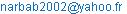(H.Norbert)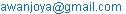(A.Wanjoya)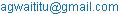(A. Waititu)

*Corresponding author

Habimana Norbert, Anthony Wanjoya, Anthony Waititu. Modeling and Forecasting Consumer Price Index (Case of Rwanda). American Journal of Theoretical and Applied Statistics. Vol. 5, No. 3, 2016, pp. 101-107. doi: 10.11648/j.ajtas.20160503.14

Received: April 10, 2016; Accepted: April 20, 2016; Published: May 3, 2016

Abstract: Consumer price index is a measure of the average change over time in the price of consumer items, goods and services that households buy for day to day living. It is one of the main indicators of economic performance and also the key indicator of the results of the monetary policy of the country, because of its wide use as a measure of inflation. The main objective of this research was to model the dynamic of CPI and to forecast its future values in the short term. Therefore, to come up with a model and forecasts of CPI, Box and Jenkins methodology were used which consists of three main steps; Model Identification, Parameter Estimation and Diagnostic Checking. Therefore, ARIMA (4,1,6) was selected as a potential model which can fits well data, as well as  to make also accurate forecast. Hence, the forecast was made for 12 months ahead of the year 2016, and the findings have shown that the CPI was likely to continue rising up with time.

Keywords: Consumer Price Index, ACF, PACF, ARIMA Model

Contents

1. Introduction

The consumer price index (CPI) is a measure of the average change over time in the price of consumer items, goods and services that households buy for day to day living. Compiled and Published by National Institute of Statistics of Rwanda every month in direct collaboration with National Bank of Rwanda. The CPI is an economic indicator and most widely used as a measure of inflation. A price index is typically assigned a value of unity, in some reference period and the values of the index for other periods of time are intended to indicate the average proportionate, or percentage, change in prices from this price reference period. Generally, it provides timely information about the rate of inflation, it is also used to adjust pensions and social security benefits in order to offset the cost of life fluctuation for the welfare of the population.

To ensure and maintain price stability are among of the main objective of National Bank of Rwanda, with a focus on price stability while taking into account of the implications of monetary policy for the economic activity and, therefore, price stability is a crucial precondition sustained economic growth. Also, it agrees on the importance of low inflation which assist businesses in making sound investment decisions, creation of jobs, protect the savings of populations and preserve the value of the national currency. In pursuing the goal of medium-term to long-term price stability, the National Bank of Rwanda also agrees with the government on the objective of keeping consumer price inflation low and stable . Therefore, CPI is one of the main indicator of economic performance and the key indicator of the results of the monetary policy of the country. Different researchers have tried to model the CPI using different techniques.

 modeled and forecasted Croatia’s CPI by using univariate seasonal ARIMA models, but precisely they wanted to examine if separate modeling and aggregating of sub-indices improves the final forecast of the all items index. Their conclusion suggested that the most precise forecasts of all items CPI are obtained by first forecasting the index’s components and then aggregating them to obtain the all items index. Also,  developed a short-term inflation forecasting by using structure time series models for each CPI component constructed at a certain level of disaggregation. Short-term forecasts of the all items CPI was made as a weighted sum of the twelve CPI components forecasts.

1.1. Statement of the Problem

Inflation is directly calculated from CPI and it is considered to be a major problem in transition economies and thus fighting inflation and maintaining stable prices is the main objective of monetary authorities like Central Bank. The negative consequences of inflation are well known, it can result in decrease in the purchasing power of the national currency leading to the aggravation of social conditions and living standards. High prices can also lead to uncertainty making domestic and foreign investors reluctant to invest in an economy . However, the update of the indicator is not always insured to be published on time, and on the other hand, to identify the factors which determines its evolution in coming months, future years, make a major problem facing decision makers and investors. And also, such information would enable the central Bank to predict the future macroeconomic development and to react in proper manner to shocks the economy is subjected to. In that context, the monetary authorities to set the monetary policy today, must necessarily justify theirs decisions based on inflation evolution in future. Therefore, the interest to resort to statistical modeling of CPI, which goes together with inflation. In this regard, the National institute of statistics of Rwanda which is responsible in producing and publishing this statistical information, should be in good position to respond to this question; "how can you describe the dynamic of CPI in Rwanda?" That is why the interest of this research, to model and forecast future value of CPI.

1.2. Justification

CPI is probably one of the most important indicator available in an economy, and many other indicators derive most of their value from the predictive ability of the CPI like inflation. And also, inflation is well known as a fragile indicator to be taken care of in any economy around the world, for the catastrophic damage that it may cause to the country when ignored. Therefore, the aim of this project is to model and forecast the future value of CPI which will be of great significant to policy makers, investors and also to the Central Bank in setting consequently monetary policies.

2. Methodology

2.1. Moving Average (MA) Models

It is one of the approach used in modeling univariate time series data, where the observed time series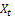depends on a weighted linear sum of past random chocks. Hence, the process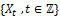is said to be a moving average of order q if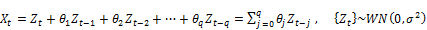(1)

Whereare constants, and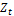are random white noise.

In fact, a MA model corresponds simply to a linear regression of the current value of the series against the random shocks of one or more prior values of the series. The disadvantages of fitting a moving average model is that it depends only on the error terms that are not observable, and the past output are not considered.

2.2. Autoregressive (AR) Models

Another approach used in modeling univariate time series is Autoregressive model, where the current observed value is assumed to be a function of past values plus a random chock. The process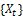is said to be an autoregressive of order p, denoted by AR (p) if,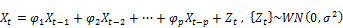(2)

So an autoregressive model corresponds simply to a linear regression of the current value of the series against one or more prior values of the series. Therefore, one can determine easily the current output, but the weakness of Autoregressive model is that the past disturbances are not considered.

2.3. ARMA Models

Another useful model of time series is formed by combining MA and AR processes. An ARMA model consists according to his name of two components: the weighted sum of past values (autoregressive component) and the weighted sum of past errors (moving average component). Then, the processis an ARMA (p,q) process if,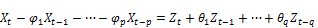(3)

Using Backward Shift Operator, the above equation can be written as,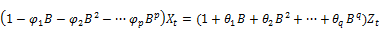Hence the more concise form,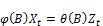(4)

With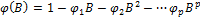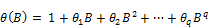The stationarity of ARMA process depends on AR part only, and the invertibility depends on MA part only. Therefore, the process will be stationary if the root of the equation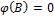lies outside unit circle. And the process is said to be invertible if the root of the equation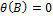lies outside unit circle.

2.4. ARIMA Models

The class of ARMA models has been already discussed for representing stationary series. So if the series is not stationary Box and Jenkins recommend differencing the time-series to achieve stationarity. Doing so produces a so-called ARIMA model, where the acronym "I" stands for integrated.

The model has the form;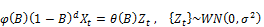(5)

Where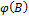andare polynomials of degrees p and q respectively and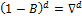is the differencing operator and d represent the order of differencing the series.

2.5. Box and Jenkins Methodology

After describing various time series models, Statisticians George Box and Jenkins developed a practical approach to build ARIMA model, precisely concerning about how to select an appropriate model that can produce accurate forecast based on a description of historical pattern in the data and how to determine the optimal model orders which best fit to a given time series and also satisfying the parsimony principle. The Box-Jenkins methodology does not assume any particular pattern in the historical data of the series to be forecasted. Rather, it uses a three step iterative approach of model identification, parameter estimation and diagnostic checking to determine the best parsimonious model from a general class of ARIMA model. This three-step process is repeated several times until a satisfactory model is finally selected. Then this model can be used for forecasting future values of the time series.

2.5.1. Model Identification

Once the series is confirmed to be stationary, one may proceed by tentatively choosing the appropriate order of models through visual inspection of plots, both the Autocorrelation Function (ACF) and Partial Autocorrelation Functions (PACF).

The relevant properties are set out as follows;

The series exhibit an AR (p) process, if the ACF decays exponentially (either direct or oscillatory) and PACF cut off after lag p.

The series exhibit a MA (q) process, if the PACF decays exponentially (either direct or oscillatory) and ACF cut off after lag q.

The series exhibit an ARMA (p, q) process, if the PACF decays exponentially (either direct or oscillatory) and ACF decays exponentially (either direct or oscillatory)

2.5.2. Parameter Estimation

The parameters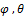and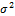are estimated by the conditional maximum likelihood, and it is supposed that the errors are independent and normally distributed.

The joint probability density of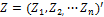is given by;(6)

Let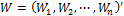be the transformed series and assume the initial conditions,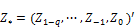The likelihood function of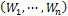given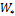andis given by;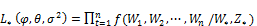(7)

The conditional likelihood function is expressed as follows;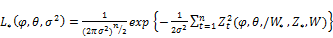(8)

The maximum likelihood suggest that we maximize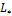with respect to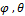and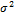.

Where the logarithm is introduced to get the conditional log-likelihood;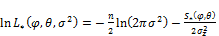(9)

Where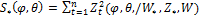In the conditional sum of squares function, the quantities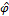andwhich maximize the log-likelihood are called the conditional maximum likelihood estimators.

The estimators that maximize the conditional likelihood function are the same which minimize the conditional sum of squares function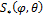which we note doesn't contain the parameter.

Once the values ofandhave been estimated (these do not depend on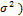the valueis obtained by maximizingwith respect to.

For fixed values of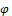and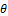we get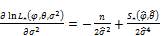(10)

Hence2.5.3. Diagnostic Checking

Once a model has been fitted, the final step is the diagnostic checking whether the model assumptions are satisfied. The basic assumption is that the residuals behave like white noise, where the errors are uncorrelated with zero mean and constant variance.

To test the autocorrelation of residuals Box-Ljung Test is used, and its statistic is defined as follows;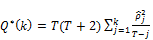(11)

The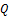statistic is asymptoptically distributed as a Chi-square distribution2.6. Forecasting

Once a model has been fitted to the data, one can make forecast future values of time series. Knowing the past until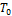the forecast of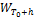is given by;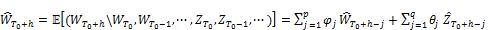(12)

Actually,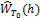is obtained from the model equation by replacing the future value ofby zero, the future value of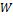by their conditional expectation and past values ofand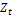by their observed values.

The forecast error variance is given by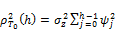(13)

Therefore, as long as the forecasts errors are normally distributed, a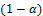probability interval for the forecasts values can be constructed as,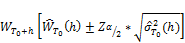(14)

2.7. Forecasting Accuracy

From the difference of forecasted and observed value, the following measures were used to assess the accuracy of the model. MAE (Mean Absolute Error), RMSE (Root Mean Squared Error) and MAPE (Mean Absolute Percentage Error).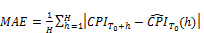(15)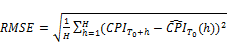(16)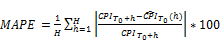(17)

3. Results and Discussions

The monthly values of consumer price index (CPI) that were used for this research are expressed in Rwandan currency, and are covering the period from February 1995 to December 2015 which makes a total of 251 observations. Therefore, the unity of time is a month, and the data from February 1995 to December 2013 were used for model fitting and those from January 2014 to December 2015 were used for accessing the forecasting power. The data were obtained from the Central Bank of Rwanda database and are also published on monthly bulletin of economic indicators at the Bank website.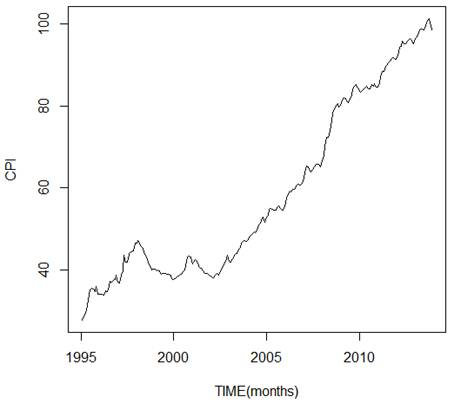Figure 1. CPI Evolution (1995-2013).

From the Figure 1, the plot shows a general increment of the CPI which leads also to the inflation explained by the depreciation of the currency. The long-term increase of data represents a trend, which explains also that the mean is not constant and depends on time. For that reason, if the mean seems obviously to be not constant, hence the series is not stationary. Therefore, the series must be transformed for any further statistical inference. Again, by visual inspection of the plot, there is no periodic fluctuation hence no seasonal component from the series.

Stationarity is tested by checking the presence or absence of unit root, where the null hypothesis is stated by default as the presence of unit root where the series is not stationary. The test from Augmented Dickey-Fuller (ADF) was used and gave the value of -0.87013 and the p-value of 0.9542 respectively. As the p-value is greater than 5% significance level, we fail to reject the null hypothesis hence the series is not stationary.

After first differencing of the original series, from the Figure 2. It can be seen that there is no systematic increase or decrease of the trend, where the mean looks to be constant over time. Again, the test from the Dickey Fuller with a p-value of 0.01 at 5% significance level, we reject the null hypothesis and confirm the stationarity of the series after first integration.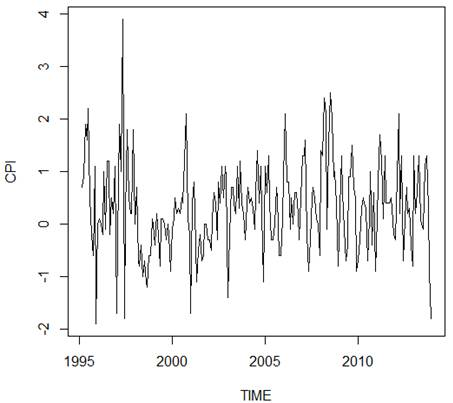Figure 2. CPI after first difference.

To identify an appropriate model, the ACF and PACF plots were used to come up with the order of the model.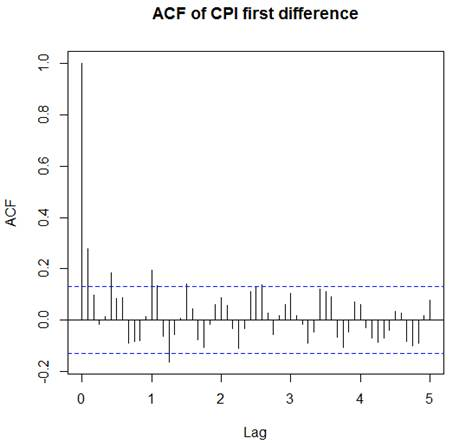Figure 3. ACF of CPI from first difference.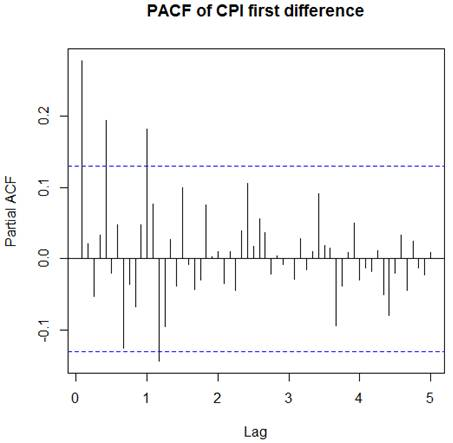Figure 4. PACF of CPI from first difference.

After the series has been stationarized by first differencing, the next step in fitting an ARIMA model is to determine how many AR or MA terms are needed to correct any autocorrelation that remains in the differenced series. Therefore, the numbers of AR and/or MA terms that are needed to fit a model are tentatively identified by looking the ACF and PACF plots of the differenced series.

If the PACF of the differenced series shows a cut off at lag k, it means that the series is still not yet fully differenced (under differenced) and then by adding enough autoregressive terms can remove any autocorrelation left from a stationarized series. The lag at which the PACF cut off tells us how many AR terms are needed. For the same case for PACF, if the ACF cut off at lag k, this indicates that exactly k MA terms that are needed to remove the remaining autocorrelation from the differenced series. Hence, by visual inspection of the Figure 3 and 4, the number of significant correlation lags from the ACF plot are almost 6 and the number of significant correlation lags from the PACF plot looks to be 4. Therefore, the first tentatively model was ARIMA (4,1,6) where p=4 & q=6 are the order of autoregressive and moving average model respectively, and d=1 is the order of integration.

The chosen model is normally the one with the least value of BIC, AIC and AICc, and satisfying also the parsimony principle which favors the least parameter possible in the model. Thus, ARIMA (4,1,3) was the one to satisfy the previous conditions with least value of AICc. Actually, the purpose of this study was to model and forecast the future value of CPI. Therefore, the model could not be only validated from Akaike Information Criteria only, as long as it is known that the model that fits well data, doesn't mean will produce accurate forecast. That's why before selecting potential models, out-of-sample forecasting accuracy must be performed to assess the model which can fits well data and which can make also accurate forecast as well.

To perform this test, the training set and test set shall be used. The training set also called "in-sample-data" is used to fit the model, and the test set also known as "out-of-sample data" is used to test the model to see how selected model may behave to new data that exist already but which were not used to fit the model.

The potential model was selected accordingly to the one which minimize most the forecasting errors like MAE, RMSE and MAPE. For that reason, two years (2014 and 2015) were used as an out-of-sample data, and data from 1995 up to 2013 were used to fit different models. The fitted models were used to forecast two years ahead, with the purpose to see how the forecasts are close to the real data (out-of-sample). Therefore, the ARIMA (4,1,6) model was the one with the smallest forecasting error comparing to the rest of other models despite their smallest value of BIC, AIC and AICc. But, before confirming the selected model, diagnostic checking was also performed to see whether the errors are uncorrelated (behave like white noise), and normally distributed.

Table 1. Estimated Parameters of ARIMA (4,1,6).

 Coefficients Estimates Standard Error t-value p-value ar1 1.2592 0.1871 6.7300 1.7151x1011 ar2 -0.5237 0.3672 -1.4261 1.5375x1001 ar3 -0.4578 0.3569 -1.2827 1.9962x1001 ar4 0.1726 0.1724 1.0011 3.5728x1005 ma1 -0.9928 0.1966 -5.0498 4.4037x1007 ma2 0.3241 0.3193 1.0150 3.1002x1001 ma3 0.4853 0.2619 1.8529 6.3882x1002 ma4 -0.6375 0.1154 -5.5242 3.3397x1008 ma5 0.062 0.095 0.6526 5.1372x1001 ma6 -0.1532 0.0637 -2.4050 1.6111x1002

As suggested by Box and Jenkins, after selecting a potential model and estimating its parameters, the next step is diagnostic checking to see if the selected model fits well the data. The main assumption is that from the fitted model, the residuals are expected to be randomly independent and identically distributed following the normal distribution. In short, they must behave like a white noise. Box-Ljung test and ACF were used to check whether the residuals are correlated. Shapiro test, histogram and normal Q-Q plot were used also for normality checking.

From the Box-Ljung test, the p-value was reported to be 0.9139 and at 5% level of significance, we failed to reject the null hypothesis of a white noise process.

From the figure 5. It can be seen on ACF plot that there are no significant spikes, all of them are within the boundaries. So, there is no residual correlation left in CPI data

Also, from Shapiro-Test for normality the test value was 0.9767 and p-value of 0.085. The null hypothesis suggest the normality of residuals, and the alternative hypothesis calls the lack of normality in residuals. Then, with 5% confidence level we fail to reject the null hypothesis.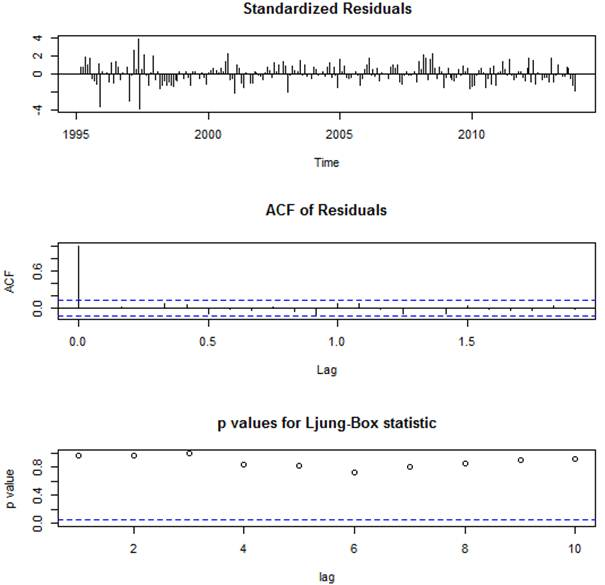Figure 5. ACF of Residuals and p-values for Box-Ljung test at different lags.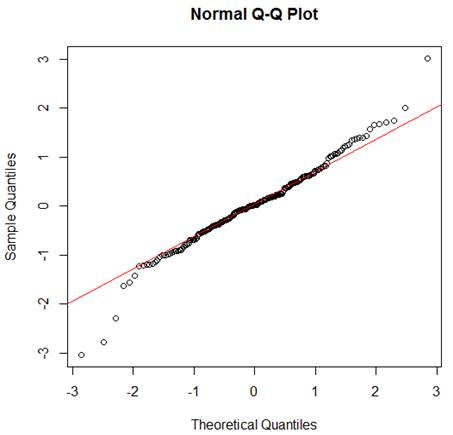Figure 6. Normal Q-Q plot of residuals.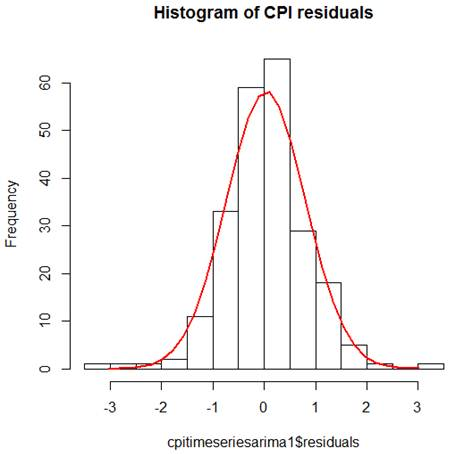Figure 7. Histogram of CPI residuals.

Figure 6 and 7 show that the residuals are almost exactly normally distributed, and it can be also confirmed that there is no correlation in the residuals which means there is no left information in the residuals to be used in fitting a model. Therefore, the ARIMA (4,1,6) was successfully selected as a potential model to be used for forecasting.

As the main objective of this study was forecasting, after successfully identifying a potential model that describes well the historical data of consumer price index, the same model were used also to forecast the future values in short term (12 months ahead).

Table 2. Future forecasts from ARIMA (4,1,6).

 Point Forecasts Lo 80 Hi 80 Lo 95 Hi 95 Jan 2016 103.594 94.447 112.740 89.606 117.582 Feb 2016 104.096 94.644 113.548 89.640 118.551 Mar 2016 104.742 94.990 114.495 89.827 119.658 Apr 2016 105.101 95.59 115.144 89.742 120.460 May 2016 105.029 94.703 115.356 89.237 120.822 Jun 2016 104.813 94.198 115.427 88.579 121.047 Jul 2016 104.874 93.960 115.789 88.182 121.567 Aug 2016 105.354 94.132 116.575 88.192 122.515 Sep 2016 105.973 94.449 117.497 88.348 123.598 Oct 2016 106.319 94.502 118.137 88.246 124.393 Nov 2016 106.256 94.150 118.361 87.741 124.770 Dec 2016 106.052 93.653 118.450 87.090 125.014

Table 3. Forecasting Evaluation.

 Statistic ME RMSE MAE MPE In sample data 0.0312 0.776 0.5742 0.06138

From table 3, the smallest value of ME indicate that the fitted model can produce accurate forecast. Also, if the value of ME is positive, the model seems to under-forecast.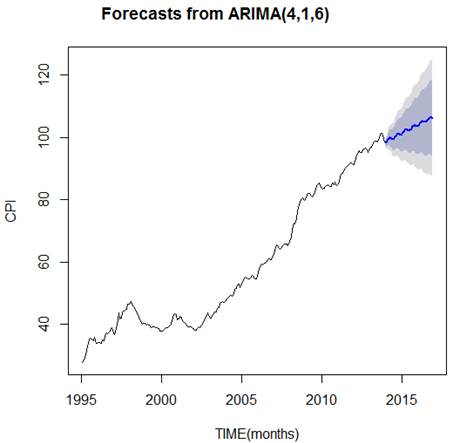Figure 8. Forecasting Evolution of CPI (January-December 2016).

From the Figure 8, it is obvious remarkable the general increment of CPI, where the future values are likely to continue rise up with time. Thereby, this means as well the increment of inflation, or in other words the continual depreciation of the currency.

4. Conclusion and Recommendation

The primary purpose behind this study was to find out which ARIMA model is more accurate and appropriate for forecasting purposes in the real world situation. Therefore, the first step was to plot the data to check if they exhibit any kind of patterns like a trend, seasonal component or any outliers. From the results, it was found a positive trend where CPI was increasing with time which leads to the non-stationarity of the series. After first differencing was applied to make a series stationary, the Autocorrelation Function (ACF) and Partial Autocorrelation Function (PACF) plots were used to choose tentatively different potential models. Among the chosen models, BIC, AIC, and AICc were used to select the best model which fits well data, by selecting the one with least value of those statistics respectively. The ARIMA (3,1,4) was selected to be the best describing the historical data of CPI, but it was not enough to conclude if it was also good for forecasting.

To assess forecasting performance, the models were used to forecast Out-of-Sample to judge the best by selecting the one which minimizes the most forecasting errors like MAE, RMSE and MAPE. Therefore, the ARIMA (4,1,6) was found to be the best among of all proposed models through different test performed to assess the goodness of fit. Box-Ljung test was used to check if the residuals behave like a white noise, and it was confirmed that the residuals were not correlated, and also via plots by visual inspection and Shapiro test the residuals were normally distributed. In fact, it has observed that the actual values of CPI (Out-of-sample data) and those predicted based on ARIMA (4,1,6) model were much closer than those of other models.

The general recommendation goes directly to the Central Bank, precisely monetary policy to be vigilant in their policy making, because this study really shows that the CPI is likely to continue rising up over time, which leads obviously to the serious inflation if nothing is done accordingly. Also, we suggest the use of Box and Jenkins methodology given its ability of describing well historical data and making good forecasts.

References

1. National Bank of Rwanda (2015), Consumer Price Index. Statistical bulletin.
2. Pufnik, A. and D. Kunovac (2006). Short-Term Forecasting of Inflation in Croatia with Seasonal ARIMA Processes. Working Paper, W-16, Croatia National Bank.
3. Mordi, C. N. O, Adeby, M. A, and Adamgbe,E.T(2012). Short-term inflation forecasting for monetary policy in Nigeria, central Bank of Nigeria Occasion Paper No. 42
4. Asel ISAKOVA (2007). Modeling and Forecasting inflation in developing Countries: The case of Economies in Central Asia. Discussion Paper No.2007-174
5. Prapanana M, Labani S. and Saptarsi G. (2014). Study of Effectiveness of Time series Modeling (ARIMA) in Forecasting Stock Prices. Internationa Journal of computer Sciences, Engineering and Application. Vol. 4, No. 2
6. Jiban Chandra P., Md Shahidul Hoque and Mohammad M. Rahman (2013). Selection of Best ARIMA Model for forecasting Average Dairly Share Price Index of pharmaceutical Companies in bangladesh: A case Study on Squaree pharmaceutical Ltd. Global Journal Inc. (USA), Volume 13
7. Ayodele A. Adebiyi, Aderemi O. Adewumi and Charles K. Ayo (2014). Stock Price Prediction Using ARIMA Model. International conference on Computer Modelling and simulation. No 16
8. Brockwell, P. J and Davis, A. R. (2002). Introduction to time Series and forecasting, Second Edition, Springer Verlag.
9. Fuller, W. A. (1996). Introduction to Statistical Time Series. John Wiley & Sons.
10. Ngai hang C. (2002). Time series Application to Finance. John Wiley & Sons.
11. S. O. Adams, A. Awujola, A. I. Alumgudu (2014). Modeling Nigeria’s Consumer Price index using ARIMA model. International Journal of Development and Economic Sustainability. Vol. 2, No. 2, pp. 37-47, June 2014.
12. Suleman, N. and S. Sarpong (2012). Empirical Approach to Modeling and Forecasting Inflation in Ghana. Current Research Journal of Economic Theory Vol. 4, no 3: 83-87.
13. Sani I. Dogua and Sarah O. Alade (2013). Short-Term Inflation Forecasting for Nigeria. CBN\ Journal of Applied Statistics Vol. 4 No.2
14. Tahsina Akhter (2013). Short-Term Forecasting of Inflation in Bangladesh with Seasonal ARIMA Processes. Munich Personal RePEc Archive (MPRA) Paper No. 43729.
15. Abraham and Katharine G., (1997). The CPI commission: Discussion, American Economic Review, May 1997.
16. www.bls.gov/opub/hom/pdf/homch17.pdf. Consulted on 25 june 2015.
17. National Institute of Statistics of Rwanda (2015). CPI Publication.

 Contents 1. 1.1. 1.2. 2. 2.1. 2.2. 2.3. 2.4. 2.5. 2.6. 2.7. 3. 4.
Article ToolsAbstractPDF(1102K)